• 强化学习的一些相关概念 智能体（Agent）： 智能体对环境进行观察，决策出行动，获得一个从环境返回的奖励 决策（Decision）：意识层面的 行动（Action ， a）：物质层面的 环境（Environment）：与智能体交互的...
————————————————————2020.8.11更新————————————————————
强化学习的一些相关概念

智能体（Agent）： 智能体对环境进行观察，决策出行动，获得一个从环境返回的奖励
决策（Decision）：意识层面的
行动（Action ， a）：物质层面的
环境（Environment）：与智能体交互的对象
状态（State，s）：是历史信息的函数，包含所有已有的信息。
奖励（Reward，R）：是智能体采取行动后环境的一个反馈
策略（Policy）：是状态到动作的函数
价值函数（Value function）：是评价状态的一个指标
模型（Model）：是个体对环境的建模

例题 1
背景描述：假设某个楼层共有 5 个房间，房间之间通过一道门相连，正如下图所示。我们将
房间编号为房间 0 到房间 4，楼层的外部，编号为 5。注意到房间 1 和房间 4 都可以直接通
到外部 5。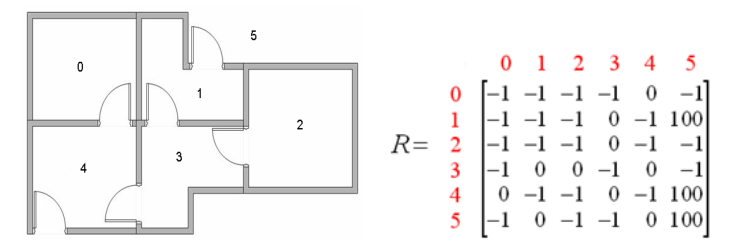问题：假设有一个机器人对楼层的布局事先不知道，如何让一个机器人，从任意房间出发，能走到外面吗？
从奖励矩阵 R 出发来理解各个概念：
智能体（Agent）：机器人
决策（Decision）：从当前所在房间准备往哪里去
行动（Action）：决策好到哪里去后，行动到那里去
环境（Environment）：楼层布局
状态（State）：当前所在房间
奖励（Reward）：从当前房间走到另一个房间的奖励值（奖励矩阵中-1 表示无效值）
奖励矩阵 R(state, action)或 R(s, a): 每个元素值 R(s,a)表示在当前状态 s 下，采取行动 a 后
的即时奖励。
Q-学习简介
Q 矩阵：跟 R 矩阵维数相同，不同的是 Q(s, a)表示在当前状态 s 下，采取行动 a 后的后续累计奖励。是状态 s 和行动 a的函数， Q(s, a)用于评价当前状态 s 下，采取行动 a的结果好坏，Q也叫动作价值函数
假定已经获得一个最优的 Q 矩阵，则对任意状态 s 出发，到达目标状态的算法如下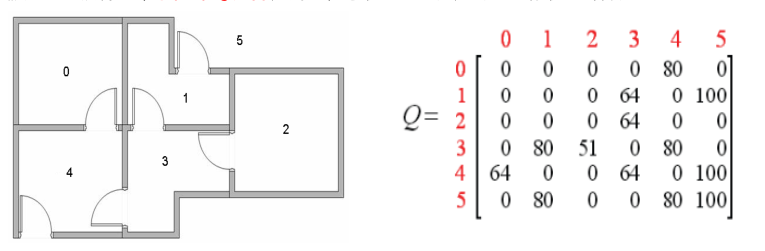步骤 1：随机选择一个初始状态 s
步骤 2：确定行动 a，使它满足 a = argmax{  Q(s , $\widetilde{a}$)}，$\widetilde{a}$∈A ，A是所有可能行动集合
步骤 3：令 s = $\widetilde{s}$，（$\widetilde{s}$为执行动作 a 后对应的下一个状态）
步骤 4：返回步骤 2
假定初始状态为 2，根据最优 Q 矩阵，有 2→3→1(4) →5→5；
假定初始状态为 1，根据最优 Q 矩阵，有 1→5→5；
假定初始状态为 0，根据最优 Q 矩阵，有 0→4→5→5；
如何获取最优的 Q 矩阵？
答案：Q-学习算法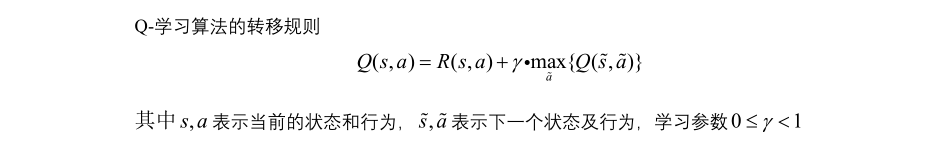Q-学习算法流程
步骤 1：给定奖励矩阵 R 和学习参数 γ，初始化 Q = 0，
步骤 2：随机选择一个初始状态 s，在当前状态 s 的所有可能行动中选取一个行动 a
步骤 3：利用选定的行为 a ， 得到下一个状态 $\widetilde{s}$
步骤 4：按照转移规则，计算Q（s，a）
步骤 5：s = $\widetilde{s}$，判断得到的Q矩阵是否收敛，不收敛则返回步骤 2，收敛则退出，完成q矩阵的学习
matlab算法如下
%%%%%%%%%%%%%%%%%%%%%%%%%%%%%%%%%%%%%%%%%%%%%%
% 机器人如何从五个房间中的任意一个走出来？
% 中南大学 自动化学院 智能控制与优化决策课题组
%%%%%%%%%%%%%%%%%%%%%%%%%%%%%%%%%%%%%%%%%%%%%
% Q学习算法
% function Q = Reinforcement_Learning(R,gamma)
clear all
clc;
format short    %显示4位小数
format compact  %压缩空格

% 输入: R and gamma
% R：即时奖励矩阵; 行、列表示状态
% -1 = 表示无效值
R = [-1,-1,-1,-1,0, -1;
-1,-1,-1,0,-1, 100;
-1,-1,-1,0,-1, -1;
-1, 0, 0,-1,0, -1;
0,-1,-1,0,-1, 100;
-1,0, -1,-1,0, 100];
gamma = 0.80;            % 学习参数

N = size(R,1); % R的行数，所有状态的个数，一行代表一个状态的所有信息
Q  = zeros(size(R));     % 初始化Q矩阵，其行数和列数和R一样。Q初始全是0
Q1 = ones(size(R))*inf;  % 前一次比较对象，判定收敛，Q初始全是无穷大
count = 0;               % 计数器

for episode = 0:50000
% 产生随机初始状态
state = ceil(N*rand);    %产生1-N之间的随机数
% 从当前状态出发，选择一个行动
x = find(R(state,:)>=0);   % 所有可能行动,R(state,:)代表R的第state行,find(R(state,:)>=0)代表R的第state行里面大于0的数的列位置集合，是个行向量，
%上一句代码理解成找出当前状态可以向那些状态转移
if ~isempty(x)   %如果有可以转移的
x1= x((ceil(length(x)*rand)));                  % 随机选择一个行动，理解成随机向一个状态转移，x1代表第几行（即为第几个状态）
end

qMax = max(Q,[],2);   %返回矩阵中每行的最大值，是一个列向量，大小是Q的行数，每行最大值物理含义是这个状态下的最好奖励（最好出路）
Q(state,x1) = R(state,x1) + gamma*qMax(x1);   % 转移规则。qMax(x1)代表x1这个状态下的最好奖励

% 判定是否收敛  其实就是前后两个Q矩阵的差很小就认为是收敛了
if sum(sum(abs(Q1-Q)))<0.0001 && sum(sum(Q>0)) %sum(sum(Q>0))代表Q大于0的数的个数,sum(sum(abs(Q1-Q)))代表Q1与Q对应位置相减绝对值之和
if count > 1000         %且学习了超过1千次
disp(strcat('强化学习的总次数： ',num2str(episode)));
break                %跳出for循环
else                    %没有学习了超过1千次
count = count+1;
end
else  %不收敛，差距很大
Q1 = Q;  %把最新的一个Q作为比较对象，覆盖掉原来的
count = 0;
end
end

% 归一化
Qmax = max(max(Q));%max(max(Q))代表Q里面最大的元素，max(Q)代表每一列最大的元素
if Qmax >0
Q = 100*Q/Qmax;
end
Q

本文来源于中南大学 自动化学院 智能控制与优化决策课题组周晓君老师的学习笔记，加了一些我自己对代码的注释，
还有两个问题未解决
1、Q-学习算法的转移规则可以换成其他的公式来产生新解迭代吗
2、除了Q-学习算法还可以用其他方法来解决Q矩阵的求取吗？
继续学强化学习，看看有啥新的体会与想法没
强化学习学习什么东西？
1、Q矩阵，动作价值函数算出来的一个矩阵，其中的Q（s，a）评价当前状态 s 下，采取行动 a 后的结果好坏程度。
2、策略函数π，π（s，a）代表当前状态 s 下，采取行动 a 的一个概率。当前状态下所有行动的概率和为1.
只要学到其中一个就可以实现对智能体的控制了。上面代码显示的就是第一种——Q矩阵的学习


展开全文• Q学习算法怎样用C++代码实现呢？？可以执行的源代码，，谢谢了，，技术小白c++ 代码 技术 源代码
• ## 【强化学习】Q-Learning算法详解

万次阅读 多人点赞 2018-06-19 21:18:18
QLearning是强化学习算法中值迭代的算法，Q即为Q（s,a）就是在某一时刻的 s 状态下(s∈S)，采取 a (a∈A)动作能够获得收益的期望，环境会根据agent的动作反馈相应的回报reward r，所以算法的主要思想就是将State与...
【强化学习】Q-Learning详解

https://morvanzhou.github.io/tutorials/machine-learning/reinforcement-learning/2-1-general-rl/ 莫凡大神的有趣的强化学习视频通俗易懂

发现了很多RL资料搬砖过来，刚入门的可以用得上

David Silver 博士的 UCL 公开课：http://www0.cs.ucl.ac.uk/staff/d.silver/web/Teaching.html
Sergey Levine 的DRL课程：http://rail.eecs.berkeley.edu/deeprlcourse/
OpenAI 的 Spinning Up in Deep RL：https://blog.openai.com/spinning-up-in-deep-rl/
关于深度强化学习良心paper：https://arxiv.org/abs/1810.06339

1、算法思想
QLearning是强化学习算法中value-based的算法，Q即为Q（s,a）就是在某一时刻的 s 状态下(s∈S)，采取 动作a (a∈A)动作能够获得收益的期望，环境会根据agent的动作反馈相应的回报reward r，所以算法的主要思想就是将State与Action构建成一张Q-table来存储Q值，然后根据Q值来选取能够获得最大的收益的动作。

Q-Table
a1
a2

s1
q(s1,a1)
q(s1,a2)

s2
q(s2,a1)
q(s2,a2)

s3
q(s3,a1)
q(s3,a2)

2、公式推导
举个例子如图有一个GridWorld的游戏从起点出发到达终点为胜利掉进陷阱为失败。智能体（Agent）、环境状态（environment）、奖励（reward）、动作（action）可以将问题抽象成一个马尔科夫决策过程，我们在每个格子都算是一个状态 $s_t$ , π(a|s)在s状态下采取动作a策略 。 P(s’|s,a)也可以写成$P_{ss'}^a$为在s状态下选择a动作转换到下一个状态s’的概率。R(s’|s,a)表示在s状态下采取a动作转移到s’的奖励reward，我们的目的很明确就是找到一条能够到达终点获得最大奖赏的策略。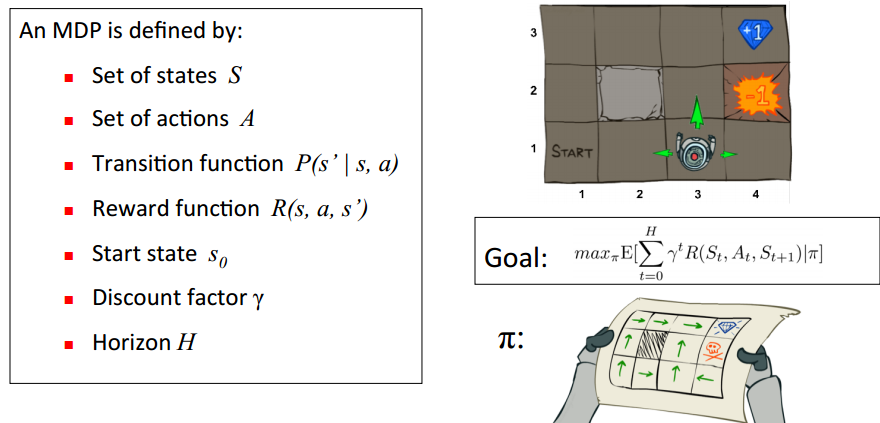所以目标就是求出累计奖励最大的策略的期望：
Goal：$\max_πE[\sum_{t=0}^{H}γ^tR(S_t,A_t,S_{t+1}) | π]$
Qlearning的主要优势就是使用了时间差分法TD（融合了蒙特卡洛和动态规划）能够进行离线学习, 使用bellman方程可以对马尔科夫过程求解最优策略
贝尔曼方程
通过bellman方程求解马尔科夫决策过程的最佳决策序列，状态值函数$V_\pi(s)$可以评价当前状态的好坏，每个状态的值不仅由当前状态决定还要由后面的状态决定，所以状态的累计奖励求期望就可得出当前s的状态值函数V(s)。bellman方程如下
$V_π(s) = E(U_t|S_t = s)$
$V_π(s) = E_π[R_{t+1}+γ[R_{t+2} + γ[.......]]|S_t = s]$
$V_π(s) = E_π[R_{t+1}+γV(s')|S_t = s]$
最优累计期望可用$V^*(s)$表示，可知最优值函数就是$V^*(s)=max_πV_\pi(s)$
$V^*(s)=\max_πE[\sum_{t=0}^{H}γ^tR(S_t,A_t,S_{t+1}) | π,s_0=s]$
Q(s,a)状态动作值函数
$q_π(s,a) = E_π[r_{t+1}+γr_{t+2}+γ^2r_{t+3}+....|A_t=a,S_t=s]$
$q_π(s,a) = E_π[G_t|A_t=a,S_t=s]$
其中$G_t$是t时刻开始的总折扣奖励，从这里我们能看出来 γ衰变值对Q函数的影响，γ越接近于1代表它越有远见会着重考虑后续状态的的价值，当γ接近0的时候就会变得近视只考虑当前的利益的影响。所以从0到1，算法就会越来越会考虑后续回报的影响。
$q_π(s,a) = E_π[R_{t+1}+γq_π(S_{t+1},A_{t+1})|A_t=a,S_t=s]$
最优价值动作函数$Q^*(s,a)=max_\pi Q^*(s,a)$,打开期望如下
$Q^*(s,a)=\sum_{s'} P(s'|s,a)(R(s,a,s')+γ\max_{a'}Q^*(s',a'))$
Bellman方程实际上就是价值动作函数的转换关系
$V_π(s) = \sum_{a∈A}π(a|s)q_π(s,a)$
$q_π(s,a) = R_s^a + γ\sum_{s'∈S}P_{ss'}^aV_π(s')$
$V_π(s)=\sum_{a'∈A}π(a|s)[R_s^a+γ\sum_{s'}P_{ss'}^aV_π(s')]$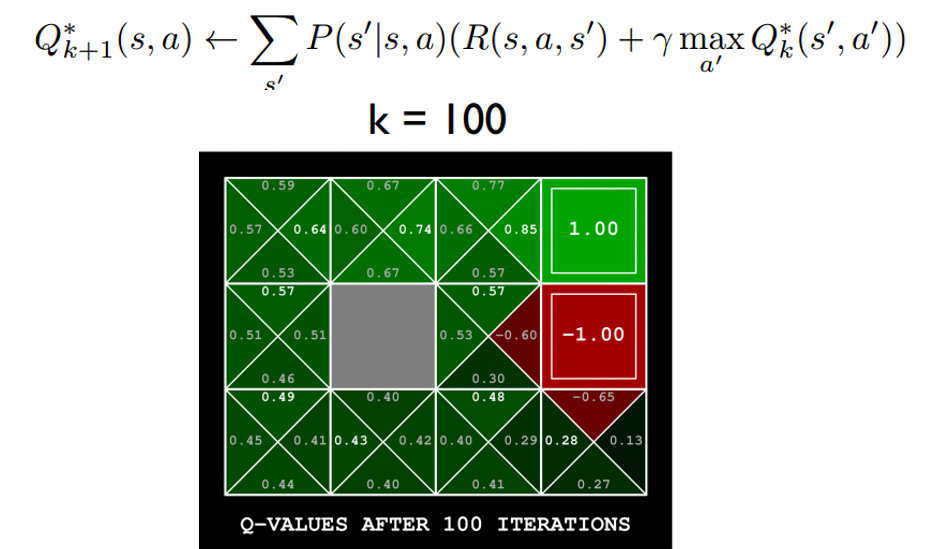根据下图更直观的了解V(s)与Q(s,a)的关系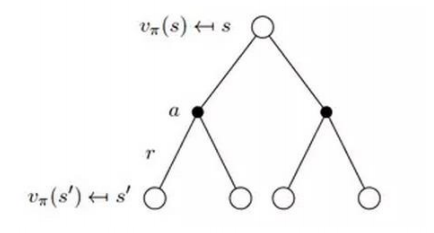时间差分法 https://blog.csdn.net/qq_30615903/article/details/80821061
时间差分方法结合了蒙特卡罗的采样方法和动态规划方法的bootstrapping(利用后继状态的值函数估计当前值函数)使得他可以适用于model-free的算法并且是单步更新，速度更快。值函数计算方式如下
$V(s)←V(s)+\alpha (R_{t+1}+\gamma V(s')-V(s))$
其中$R_{t+1}+\gamma V(s')$被称为TD目标，$\delta_t=R_{t+1}+\gamma V(s')-V(s)$  称为TD偏差。
3、更新公式
根据以上推导可以对Q值进行计算，所以有了Q值我们就可以进行学习，也就是Q-table的更新过程，其中α为学习率γ为奖励性衰变系数，采用时间差分法的方法进行更新。
$Q(s,a) ← Q(s,a) + α[r + γmax_{a'}Q(s',a')-Q(s,a)]$
上式就是Q-learning更新的公式，根据下一个状态s’中选取最大的$Q(s',a')$值乘以衰变γ加上真实回报值最为Q现实，而根据过往Q表里面的Q(s,a)作为Q估计。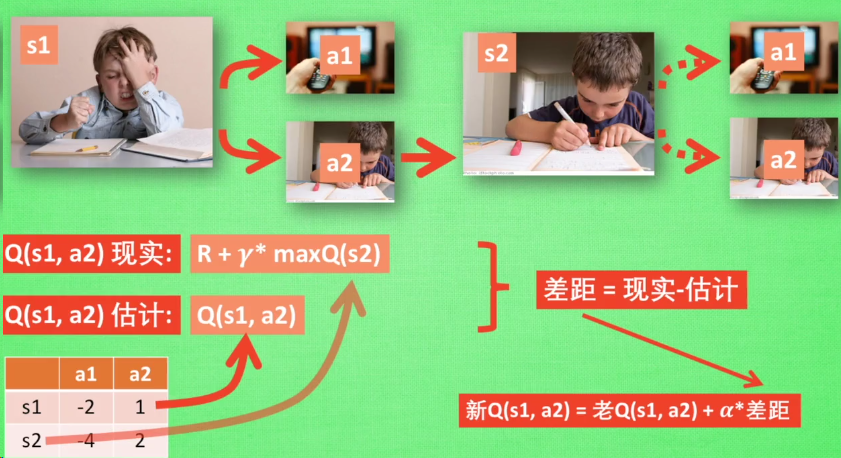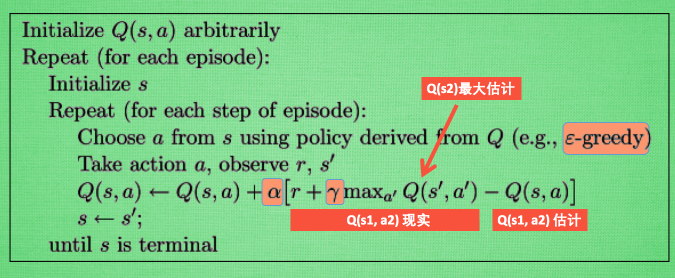4、实现代码
代码来自网上各路大神的源码，非原创，据反映没图片跑不通，所以建了个github，https://github.com/xshura/reinforcement_learning
Q-Learning agent
# -*- coding: utf-8 -*-
import random
from environment import Env
from collections import defaultdict

class QLearningAgent:
def __init__(self, actions):
# actions = [0, 1, 2, 3]
self.actions = actions
self.learning_rate = 0.01
self.discount_factor = 0.9
self.epsilon = 0.1
self.q_table = defaultdict(lambda: [0.0, 0.0, 0.0, 0.0])

# 采样 <s, a, r, s'>
def learn(self, state, action, reward, next_state):
current_q = self.q_table[state][action]
# 贝尔曼方程更新
new_q = reward + self.discount_factor * max(self.q_table[next_state])
self.q_table[state][action] += self.learning_rate * (new_q - current_q)

# 从Q-table中选取动作
def get_action(self, state):
if np.random.rand() < self.epsilon:
# 贪婪策略随机探索动作
action = np.random.choice(self.actions)
else:
# 从q表中选择
state_action = self.q_table[state]
action = self.arg_max(state_action)
return action

@staticmethod
def arg_max(state_action):
max_index_list = []
max_value = state_action
for index, value in enumerate(state_action):
if value > max_value:
max_index_list.clear()
max_value = value
max_index_list.append(index)
elif value == max_value:
max_index_list.append(index)
return random.choice(max_index_list)

if __name__ == "__main__":
env = Env()
agent = QLearningAgent(actions=list(range(env.n_actions)))
for episode in range(1000):
state = env.reset()
while True:
env.render()
# agent产生动作
action = agent.get_action(str(state))
next_state, reward, done = env.step(action)
# 更新Q表
agent.learn(str(state), action, reward, str(next_state))
state = next_state
env.print_value_all(agent.q_table)
# 当到达终点就终止游戏开始新一轮训练
if done:
break

环境部分
import time
import numpy as np
import tkinter as tk
from PIL import ImageTk, Image

np.random.seed(1)
PhotoImage = ImageTk.PhotoImage
UNIT = 100
HEIGHT = 5
WIDTH = 5

class Env(tk.Tk):
def __init__(self):
super(Env, self).__init__()
self.action_space = ['u', 'd', 'l', 'r']
self.n_actions = len(self.action_space)
self.title('Q Learning')
self.geometry('{0}x{1}'.format(HEIGHT * UNIT, HEIGHT * UNIT))
self.canvas = self._build_canvas()
self.texts = []

def _build_canvas(self):
canvas = tk.Canvas(self, bg='white',
height=HEIGHT * UNIT,
width=WIDTH * UNIT)
# create grids
for c in range(0, WIDTH * UNIT, UNIT):  # 0~400 by 80
x0, y0, x1, y1 = c, 0, c, HEIGHT * UNIT
canvas.create_line(x0, y0, x1, y1)
for r in range(0, HEIGHT * UNIT, UNIT):  # 0~400 by 80
x0, y0, x1, y1 = 0, r, HEIGHT * UNIT, r
canvas.create_line(x0, y0, x1, y1)

self.rectangle = canvas.create_image(50, 50, image=self.shapes)
self.triangle1 = canvas.create_image(250, 150, image=self.shapes)
self.triangle2 = canvas.create_image(150, 250, image=self.shapes)
self.circle = canvas.create_image(250, 250, image=self.shapes)

# pack all
canvas.pack()

return canvas

rectangle = PhotoImage(
Image.open("../img/rectangle.png").resize((65, 65)))
triangle = PhotoImage(
Image.open("../img/triangle.png").resize((65, 65)))
circle = PhotoImage(
Image.open("../img/circle.png").resize((65, 65)))

return rectangle, triangle, circle

def text_value(self, row, col, contents, action, font='Helvetica', size=10,
style='normal', anchor="nw"):
if action == 0:
origin_x, origin_y = 7, 42
elif action == 1:
origin_x, origin_y = 85, 42
elif action == 2:
origin_x, origin_y = 42, 5
else:
origin_x, origin_y = 42, 77

x, y = origin_y + (UNIT * col), origin_x + (UNIT * row)
font = (font, str(size), style)
text = self.canvas.create_text(x, y, fill="black", text=contents,
font=font, anchor=anchor)
return self.texts.append(text)

def print_value_all(self, q_table):
for i in self.texts:
self.canvas.delete(i)
self.texts.clear()
for i in range(HEIGHT):
for j in range(WIDTH):
for action in range(0, 4):
state = [i, j]
if str(state) in q_table.keys():
temp = q_table[str(state)][action]
self.text_value(j, i, round(temp, 2), action)

def coords_to_state(self, coords):
x = int((coords - 50) / 100)
y = int((coords - 50) / 100)
return [x, y]

def state_to_coords(self, state):
x = int(state * 100 + 50)
y = int(state * 100 + 50)
return [x, y]

def reset(self):
self.update()
time.sleep(0.5)
x, y = self.canvas.coords(self.rectangle)
self.canvas.move(self.rectangle, UNIT / 2 - x, UNIT / 2 - y)
self.render()
# return observation
return self.coords_to_state(self.canvas.coords(self.rectangle))

def step(self, action):
state = self.canvas.coords(self.rectangle)
base_action = np.array([0, 0])
self.render()

if action == 0:  # up
if state > UNIT:
base_action -= UNIT
elif action == 1:  # down
if state < (HEIGHT - 1) * UNIT:
base_action += UNIT
elif action == 2:  # left
if state > UNIT:
base_action -= UNIT
elif action == 3:  # right
if state < (WIDTH - 1) * UNIT:
base_action += UNIT

# 移动
self.canvas.move(self.rectangle, base_action, base_action)
self.canvas.tag_raise(self.rectangle)
next_state = self.canvas.coords(self.rectangle)
# 判断得分条件
if next_state == self.canvas.coords(self.circle):
reward = 100
done = True
elif next_state in [self.canvas.coords(self.triangle1),
self.canvas.coords(self.triangle2)]:
reward = -100
done = True
else:
reward = 0
done = False

next_state = self.coords_to_state(next_state)
return next_state, reward, done

# 渲染环境
def render(self):
time.sleep(0.03)
self.update()



展开全文• ## Q-Learning算法学习

万次阅读 2017-07-28 11:54:25
Q-Learning算法学习简介Q-Learning算法下，目标是达到目标状态(Goal State)并获取最高收益，一旦到达目标状态，最终收益保持不变。因此，目标状态又称之为吸收态。Q-Learning算法下的agent，不知道整体的环境，知道...
Q-Learning算法学习

简介

Q-Learning算法下，目标是达到目标状态(Goal State)并获取最高收益，一旦到达目标状态，最终收益保持不变。因此，目标状态又称之为吸收态。

Q-Learning算法下的agent，不知道整体的环境，知道当前状态下可以选择哪些动作。

通常，我们需要构建一个即时奖励矩阵R，用于表示从状态s到下一个状态s’的动作奖励值。

由即时奖励矩阵R计算得出指导agent行动的Q矩阵。

Q矩阵是agent的大脑。

初始时，Q矩阵元素全部初始化为0，表示当前的agent大脑一片空白，什么也不知道。

而计算Q(s,a)<!--//--><![CDATA[//><!--
Q(s,a)
//--><!]]>的推导公式是：

Q(s,a)=R(s,a)+γmax[Q(s′,all actions)]<!--//--><![CDATA[//><!--
Q(s,a) = R(s,a) + \gamma  max[Q(s',all \ actions)]
//--><!]]>

其中，s′<!--//--><![CDATA[//><!--
s'
//--><!]]>表示下一个状态。

通过这个推导公式计算出Q矩阵的元素，注意右边的max内的Q值通过查找当前的Q矩阵得到，左边是计算。而不是我们第一眼看过去理解的那样：需要用动态规划来求解。

Q-Learning算法核心

以一个episode为一个训练周期：从初始状态到终结态。

每学完一个episode后，再进入下一个episode学习。

因此，可以得到Q-Learning外层循环是一个episode，内层循环是episode的每一个step。

算法核心：

设置好 γ<!--//--><![CDATA[//><!--
\gamma
//--><!]]> 值以及矩阵R
初始化矩阵Q全为0
For each episode: Select a random initial state
Do while the goal state hasn’t been reached. Select one among all possible actions for current state
Using this possible action, consider going to the  next state
Get maximum Q value for this next state based on all possible actions
Compute: Q(s,a)=R(s,a)+γmax[Q(s′,all actions)]<!--//--><![CDATA[//><!--
Q(s,a) = R(s,a) + \gamma max [Q(s', all \ actions)]
//--><!]]>
Set the next state as the current state
End Do
End For
每个episode是一个training session，且每一轮训练的意义是：加强大脑，表现形式是agent的Q矩阵的元素更新。

当Q习得后，可以用Q矩阵来指引agent的行动。

问题是：如何使用Q矩阵？

核心是：从Q矩阵中寻找当前状态得到的最大的总收益的行动。

具体算法如下：

Set current state = initial state
From current state, find the action with the highest Q value
Set current state = next state
Repeat 2,3, until current state = goal state
所以问题的重点是在求得Q矩阵，而利用Q矩阵是比较简单的。

最终输出的是从初始状态到目标状态的状态序列，表示从当前习得的Q矩阵下做出的最优选择。
展开全文• 深度Q学习将深度学习和强化学习相结合，是第一个深度强化学习算法。深度Q学习的核心就是用一个人工神经网络q(s,a;w),s∈S,a∈Aq(s,a;w),s∈\mathcal{S} ,a∈\mathcal{A}q(s,a;w),s∈S,a∈A 来代替动作价值函数。由于...
深度Q学习
深度Q学习将深度学习和强化学习相结合，是第一个深度强化学习算法。深度Q学习的核心就是用一个人工神经网络$q(s,a;w),s∈\mathcal{S} ,a∈\mathcal{A}$ 来代替动作价值函数。由于神经网络具有强大的表达能力，能够自动寻找特征，所以采用神经网络有潜力比传统人工特征强大得多。最近基于深度Q网络的深度强化学习算法有了重大的进展，在目前学术界有非常大的影响力。
·经验回放（experience replay）：将经验（即历史的状态、动作、奖励等）存储起来，再在存储的经验中按一定的规则采样。
·目标网络（target network）：修改网络的更新方式，例如不把刚学习到的网络权重马上用于后续的自益过程。
经验回放
V.Mnih等在2013年发表文章《Playing Atari with deep reinforcement learning》，提出了基于经验回放的深度Q网络，标志着深度Q网络的诞生，也标志着深度强化学习的诞生。
采用批处理的模式能够提供稳定性。经验回放就是一种让经验的概率分布变得稳定的技术，它能提高训练的稳定性。经验回放主要有“存储”和“采样回放”两大关键步骤。
·存储：将轨迹以$(S_t,A_t,R_{t+1},S_{t+1})$等形式存储起来；
·采样回放：使用某种规则从存储的$(S_t,A_t,R_{t+1},S_{t+1})$中随机取出一条或多条经验。
算法9给出了带经验回放的Q学习最优策略求解算法。

算法9　带经验回放的Q学习最优策略求解
1.（初始化）任意初始化参数$w$。
2.逐回合执行以下操作。
2.1　（初始化状态动作对）选择状态$S$。
2.2　如果回合未结束，执行以下操作：
2.2.1　（采样）根据$q(S,·;w)$选择动作A并执行，观测得到奖励R和新状态S’；
2.2.2　（存储）将经验$(S,A,R,S')$存入经验库中；
2.2.3　（回放）从经验库中选取经验$(S_i,A_i,R_i,S_i')$；
2.2.4　（计算回报的估计值）$Ui←Ri+γmaxaq(S_i',a;w)$；
2.2.5　（更新动作价值函数）更新$w$以减小$［U_{i} -q (Si,Ai;w)］^2（如w←w+α［U_i-q(S_i,A_i;w)］▽q(S_i,A_i;w)）$
2.2.6　$S←S'$。

经验回放有以下好处。
·在训练Q网络时，可以消除数据的关联，使得数据更像是独立同分布的（独立同分布是很多有监督学习的证明条件）。这样可以减小参数更新的方差，加快收敛。
·能够重复使用经验，对于数据获取困难的情况尤其有用。从存储的角度，经验回放可以分为集中式回放和分布式回放。
·集中式回放：智能体在一个环境中运行，把经验统一存储在经验池中。
·分布式回放：智能体的多份拷贝（worker）同时在多个环境中运行，并将经验统一存储于经验池中。由于多个智能体拷贝同时生成经验，所以能够在使用更多资源的同时更快地收集经验。
从采样的角度，经验回放可以分为均匀回放和优先回放。
·均匀回放：等概率从经验集中取经验，并且用取得的经验来更新最优价值函数。
·优先回放（Prioritized Experience Replay，PER）：为经验池里的每个经验指定一个优先级，在选取经验时更倾向于选择优先级高的经验。
T.Schaul等于2016年发表文章《Prioritized experience replay》，提出了优先回放。优先回放的基本思想是为经验池里的经验指定一个优先级，在选取经验时更倾向于选择优先级高的经验。一般的做法是，如果某个经验（例如经验i）的优先级为$p_i$，那么选取该经验的概率为经验值有许多不同的选取方法，最常见的选取方法有成比例优先和基于排序优先。
·成比例优先（proportional priority）：第i个经验的优先级为$p_i=(δ_i+ε)α$其中$δ_i$是时序差分误差，$ε$是预先选择的一个小正数，$α$是正参数。
·基于排序优先（rank-based priority）：第i个经验的优先级为其中$rank_i$是第i个经验从大到小排序的排名，排名从1开始。D.Horgan等在2018发表文章《Distributed prioritized experience replay》，将分布式经验回放和优先经验回放相结合，得到分布式优先经验回放（distributed prioritized experience replay）。经验回放也不是完全没有缺点。例如，它也会导致回合更新和多步学习算法无法使用。一般情况下，如果我们将经验回放用于Q学习，就规避了这个缺点。
带目标网络的深度Q学习
对于基于自益的Q学习，其回报的估计和动作价值的估计都和权重$w$有关。当权重值变化时，回报的估计和动作价值的估计都会变化。在学习的过程中，动作价值试图追逐一个变化的回报，也容易出现不稳定的情况。在半梯度下降中，在更新价值参数$w$时，不对基于自益得到的回报估计Ut求梯度。其中一种阻止对Ut求梯度的方法就是将价值参数复制一份得到$w$目标，在计算$U_t$时用w目标计算。基于这一方法，V.Mnih等在2015年发表了论文《Human-level control through deep reinforcement learning》，提出了目标网络（target network）这一概念。目标网络是在原有的神经网络之外再搭建一份结构完全相同的网络。原先就有的神经网络称为评估网络（evaluation network）。在学习的过程中，使用目标网络来进行自益得到回报的评估值，作为学习的目标。在权重更新的过程中，只更新评估网络的权重，而不更新目标网络的权重。这样，更新权重时针对的目标不会在每次迭代都变化，是一个固定的目标。在完成一定次数的更新后，再将评估网络的权重值赋给目标网络，进而进行下一批更新。这样，目标网络也能得到更新。由于在目标网络没有变化的一段时间内回报的估计是相对固定的，目标网络的引入增加了学习的稳定性。所以，目标网络目前已经成为深度Q学习的主流做法。
算法10给出了带目标网络的深度Q学习算法。算法开始时将评估网络和目标网络初始化为相同的值。为了得到好的训练效果，应当按照神经网络的相关规则仔细初始化神经网络的参数。

算法10　带经验回放和目标网络的深度Q学习最优策略求解
1.（初始化）初始化评估网络$q(·,·;w)$的参数w；目标网络$q(·,·;w_{目标})$的参数$w_{目标}←w$。
2.逐回合执行以下操作。
2.1　（初始化状态动作对）选择状态$S$。
2.2　如果回合未结束，执行以下操作：
2.2.1　（采样）根据$q(S,·;w)$选择动作A并执行，观测得到奖励R和新状态S’；
2.2.2　（经验存储）将经验$(S,A,R,S')$存入经验库中；
2.2.3　（经验回放）从经验库中选取一批经验$(S_i,A_i,R_i,S_i')（i∈ \mathcal{B}）$；
2.2.4　（计算回报的估计值）2.2.5　（更新动作价值函数）更新以减小2.2.6　$S←S'$；
2.2.7　（更新目标网络）在一定条件下（例如访问本步若干次）更新目标网络的权重$w_{目标}←w$。

在更新目标网络时，可以简单地把评估网络的参数直接赋值给目标网络（即$w_{目标}←w$），也可以引入一个学习率α目标把旧的目标网络参数和新的评估网络参数直接做加权平均后的值赋值给目标网络（即$w_{目标}←(1-α_{目标})w_{目标}+α_{目标}w$）。事实上，直接赋值的版本是带学习率版本在$α_{目标}=1$时的特例。对于分布式学习的情形，有很多独立的拷贝（worker）同时会修改目标网络，则就更常用学习率$α_{目标}∈(0,1)$。
双重深度Q网络
Q学习会带来最大化偏差，而双重Q学习却可以消除最大化偏差。基于查找表的双重Q学习引入了两个动作价值的估计$q^{(0)}和q^{(1)}$，每次更新动作价值时用其中的一个网络确定动作，用确定的动作和另外一个网络来估计回报。
对于深度Q学习也有同样的结论。Deepmind于2015年发表论文《Deep reinforcement learning with double Q-learning》，将双重Q学习用于深度Q网络，得到了双重深度Q网络（Double Deep Q Network，Double DQN）。考虑到深度Q网络已经有了评估网络和目标网络两个网络，所以双重深度Q学习在估计回报时只需要用评估网络确定动作，用目标网络确定回报的估计即可。所以，只需要将算法10中的更换为就得到了带经验回放的双重深度Q网络算法。
对偶深度Q网络
Z.Wang等在2015年发表论文《Dueling network architectures for deep reinforcement learning》，提出了一种神经网络的结构——对偶网络（duel network）。对偶网络理论利用动作价值函数和状态价值函数之差定义了一个新的函数——优势函数（advantage function）：对偶Q网络仍然用$q(w)$来估计动作价值，只不过这时候$q(w)$是状态价值估计$v(s;w)$和优势函数估计$a(s,a;w)$的叠加，即$q(s,a;w)=v(s;w)+a(s,a;w)$。
其中$v(w$)和$a(w)$可能都只用到了$w$中的部分参数。在训练的过程中，$v(w)$和$a(w)$是共同训练的，训练过程和单独训练普通深度Q网络并无不同之处。
不过，同一个q(w)事实上存在着无穷多种分解为v(w)和a(w)的方式。如果某个$q(s,a;w)$可以分解为某个$v(s;w)和a(s,a;w)$，那么它也能分解为$v(s;w)+c(s)$和$a(s,a;w)-c(s)$，其中$c(s)$是任意一个只和状态$s$有关的函数。为了不给训练带来不必要的麻烦，往往可以通过增加一个由优势函数导出的量，使得等效的优势函数满足固定的特征，使得分解唯一。常见的方法有以下两种：
·考虑优势函数的最大值，令使得等效优势函数$a_{等效}(s,a;w) = a(s,a;w)-\max{a(s,a;w)}$满足·考虑优势函数的平均值，令使得等效优势函数$a_{等效}(s,a;w)=a(s,a;w)-\dfrac{1}{|{A}|}\sum{a(s,a;w)}$满足展开全文• QLearning  QLearning并没有直接将这个Q值（q_target是估计值）直接赋予新的Q，而是采用渐进的方式类似梯度下降，朝...一、QLearning算法思维   二、QLearning算法更新思维   1.导入模块 from maze...
• 西瓜书上的QLearning算法与网上的版本比较：  不同点：西瓜书上的Q(x,a)就是Q表，π（x,a）就是贪婪算法选择动作的概率，这个值对应于ε。  西瓜书上的第六行的公式和另一版本的更新值公式的区别，网上版本是下...
• Q-learning是一个无模型强化学习算法Q-learning的目标是学习一个策略，它告诉agent在什么情况下应该采取什么行动。它不需要环境的模型(因此就有了“无模型”的含义)，并且它可以处理随机转换和奖励的问题，而不...
• 本文是对 http://mnemstudio.org/path-finding-q-learning-tutorial.htm 的翻译，共分两部分，第...这篇教程通俗易懂，是一份很不错的学习理解 Q-learning 算法工作原理的材料。 第一部分：中文翻译第二部分：英文原
• 前期回顾 强化学习经典算法笔记(零)：贝尔曼方程的推导 强化学习经典算法笔记(一)：价值迭代算法Value Iteration ...强化学习经典算法笔记(四)：时间差分算法Temporal Difference（Q-Learning算法） 强化学习经典算...
• 1.强化学习介绍 2.Q-Learning算法实例 3.一个Q-Learning算法的程序实现机器学习 python
• 简单的Q-learning算法实现 Q-Learning算法下，目标是达到目标状态(Goal State)并获取最高收益，一旦到达目标状态，最终收益保持不变。因此，目标状态又称之为吸收态。 Q-Learning算法下的agent，不知道...
• DQN算法由于某些环境中状态过多，通过传统Q-learning的方法，维护一张规模巨大的Q表显然是不现实的神经网络 tensorflow DQN
• 本文为《MatLab强化学习入门...如何对不同的状态均形成良好的估计呢，Q学习应运而生。 本文将主要介绍Q-Learning的一系列基本算法，包括Q-Learning、Sarsa、Sarsa-λ算法；分析网格迷宫的编程实现，并对结果进行讨论。matlab
• sarsa 和 Q-learning都是时序差分下对动作价值函数优化的单步方法，算法基本上一样，只有一点： 为了更新St的动作价值函数，需要St+1的动作价值函数，St+1具体是什么St+1是由上个episode决定了，但是St+1对应的...
• 强化学习经典算法笔记——时间差分算法 强化学习经典算法笔记(零)：贝尔曼方程的推导 强化学习经典算法笔记(一)：价值迭代算法Value Iteration 强化学习经典算法笔记(二)：策略迭代算法Policy Iteration 强化...
• DQN是一种深度学习和强化学习结合的算法，提出的动机是传统的强化学习算法Q-learning中的Q_table存储空间有限，而现实世界甚至是虚拟世界中的状态是接近无限多的（比如围棋），因此，无法构建可以存储超大状态空间的...
• 个人学习网站 Q-learning 算法描述： Sarsa 算法描述： 假设我们的 Q(s, a) 是一个 Q table ，如下图所示，该表格表示共有三个 state (状态)： s1s1s_{1} 、s2s2s_{2}、s3s3s_{3} ,每个状态都有三个可...
• 强化学习和遗传算法优胜劣汰的思想类似，通过奖惩机制不断强化好的行为，弱化坏的行为。什么是好的行为，什么是坏的行为，这跟你要解决的具体问题有关，比如路径规划问题，走距离目标点较近的路线就是好的行为，走...最短路径
• 强化学习Q-learning算法详解与应用：https://blog.xupengit.top/index.php/20171218/cid=57.html人工智能 python
• ## 数据结构与算法学习笔记

万次阅读 多人点赞 2018-09-25 13:55:49
本文是王争老师的《算法与数据结构之美》的学习笔记，详细内容请看王争的专栏。有不懂的地方指出来，我做修改。 数据结构与算法思维导图 数据结构指的是“一组数据的存储结构”，算法指的是“操作数据的一组...
• 关于Q-learning 和 Sarsa 算法, 详情参见博客 需要注意的细节 训练时 采用 ϵ\epsilonϵ 贪心算法; # 贪婪动作选择，含嗓声干扰 a = np.argmax(Q_all[s, :] + np.random.randn(1, env.action_space.n) * (1. / (i ...python
• Q-learning 是强化学习中的一种常见的算法，近年来由于深度学习革命而取得了很大的成功。本教程不会解释什么是深度 Q-learning，但我们将通过 Q-learning 算法来使得代理学习如何玩 tic-tac-toe 游戏。尽管它很简单...python
• ## 机器学习算法地图

千次阅读 多人点赞 2018-07-05 12:10:07
其它机器学习、深度学习算法的全面系统讲解可以阅读《机器学习-原理、算法与应用》，清华大学出版社，雷明著，由SIGAI公众号作者倾力打造。 书的购买链接 书的勘误，优化，源代码资源 文章《机器学习算法地图》系...人工智能
• 一般来说，在训练样本不同时给定的情况下，比起将所有的训练样本集中起来同时进行学习，把训练样本逐个输入到学习算法中，并在新的数据进来的时候马上对现在的学习结果进行更新，这样的逐次学习算法更加有效。...
• 一. 强化学习的分类 1.Model-free 和 Model-based Model-free 即机器人不知道外界环境信息，只能在...诸如Q Learning， Sarsa，Policy Gradients等算法。 Model-based 指机器人对环境有一定的了解，可以对环境...Sarsa
• Sarsa是在线(On-policy)算法Q-Learning是离线(Off-policy)算法。 关于Q-Learning与Sarsa的Python代码实现，可以参考我的github： sarsa算法 sarsa(lambda)算法 Q-Learning 算法 参考资料： ...sarsa...